# Depreciation

• Study Notes
• Discuss This Topic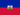Petuel E. 1 0 Why you must ascertain the key points when you're preparing a depreciation? it's to say the cost of the asset, life of the asset etc.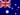Wendy C. 1 0 Depreciation is defined as the allocation of the cost of a non-current asset over the economic life of that asset. It is charged at the end of each accounting period. At the end of each accounting period depreciation accumulates and it is the 'Accumulated depreciation' account that is posted to the Balance sheet as a deduction from the relevant non-current asset.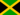Anton A. 0 0 What is depreciation?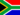Samuel F. 0 0 Is depreciation a direct or indirect cost?Samuel F. 0 0 What is the units of activity depreciation?Samuel F. 0 0 What is an asset's useful life?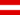Harrison A. 1 0 Depreciation is the assigning or allocating of a plant asset's cost to expense over the accounting periods that the assets is likely to be used. For example, if a business purchases a delivery truck with a cost of \$10,000 and it's expected to be used for 5 years ,the organisation might have expense of \$20,000 in each of the five years. (The amount can vary depending on the method of assumptions). Therefore, each year there will be an adjusting entry with a debit to depreciation expense for \$20,000 and a credit to accumulated depreciation for \$20,000Harrison A. 1 0 What is accumulated depreciation?Zachary B. 0 0 What does depreciation mean?Zachary B. 1 0 What does depreciation have to do with balance day adjustments?
• Text Version